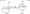# Control System January 2008

Note : 1. Answer any FIVE full questions.

2. Missing data, if any, may be suitably assumed.

1 a. Explain with examples open loop and closed loop control systems. List the merits and demerits of open loop and closed loop control systems.

b. For the mechanical system shown in fig. 1(b)

i) Draw the mechanical network ii) Write the differential equations describing the system

iii) Draw the F-V analogous electrical circuit after writing the corresponding electrical equations.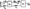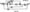Fig.2(b)

2 a. For the system shown in fig. 2(a), determine C(s)/R(s) by block diagram reduction

technique.

b. For the signal flow graph shown in the fig.2(b), obtain transfer function Y7/Yl and

y7/y2 .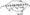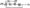Fig.3(a)

3 a. The relation of the response Y(s) to the excitation R(s) in the presence of the undesired disturbance D(s) is represented by the fig.3(a). Determine K such that, with R(s) = 0, the output Y(s) = 0 for any disturbance D(s).

b.  It is desired that a control system with unity feed back, and forward path transfer function (s + a) G(s) = be stable and the steady state error for a unit step s3 + (l + a)s2 + (a – l)s +1 – a input be less than or equal to 0.05. Determine the range of a that meets both requirements.

c.   Define the term transfer function. The unit step response of single loop, UFBCS is given by C(t)= l-i.25e~2t + 0.25e~1Ot. Determine its closed loop and open loop transfer function.         (08 Marks)

4 a. What are static error coefficients? Derive expressions for the same.

b.  Explain the following time domain specifications of a second order system:

(i) Maximum overshoot ii) Peak time iii) Delay time iv) Rise time v) Settling time.

c.  For a servomechanism system with G(s) = and H(s) = 1 + K2s, determine the value of Ki and K.2 so that the peak overshoot to a unit step response is 0.25 and peak time is 2 seconds.

5 a. Show that the root loci for UFBCS, Cj(s) – + ^Xs + 3) js a circ}ew s(s + l)

b. Draw the root locus diagram for the loop transfer function G(s)h(s) = -r-z\

s^s +8s + 17j the root locus evaluate the value of‘K’ for a system damping ratio of 0.5. From

6 a. The polynomial F(s) = s4 + 2s3 + 3s2 -f s +1 has all its roots in left hand side of a ‘s’ plane. Use R-H criterion to determine the number of roots of F(s) lying between s = -0.5 and S = -l. (10 Marks)

b.  State Routh-Hurwitz stability criterion. The system shown in fig.6(b) oscillates at a frequency of 2 radians/sec. Using R-H criterion determine the values of ‘K’ and ‘a’.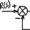Fig.6(b)

7 a. The open loop transfer function of a unity feed back control system is given by KG(s) =. Draw the asymptotic Bode plots and hence find the value ofv/ s(l + 0.02sXl + 0.05s)\ ‘K’ for which the gain margin is 10 dB. What is the corresponding phase  margin?

b.  Estimate the transfer function from the Bode plot shown in fig.7(b). Digits on line indicate slope in dB/dec.

8 a. For a feedback system with G(s) =( ,, r, H(s) = —, determine peak resonance and s(s +1 j(s + 5j resonance frequency. Derive the expressions used. Fig.8(b)(i) and Fig.8

(b)(ii) represent Nyquist plots drawn for © = 0 to co = oo for two different G(s)H(s). Neither of these have poles on RH plane. For each case i) Complete the plot for ‘oo’ values on negative imaginary axis and oo = 0+ to m — 0.. ii) Indicate the type of G(s)H(s) iii) Is the system stable? If not determine the number of zeros 1 + G(s)H(s) on